# The Namer-Claimer game

Consider the following game played on the integers fromto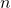.  In each round Namer names a forbidden distance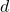, then Claimer claims a subset of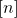that does not contain any two integers at distance.  After finitely many rounds, Claimer will have claimed sets that cover the whole of, at which point the game ends.  How many rounds will there be with best play?

I’ve run this question out at a number of workshops and open problems sessions, and haven’t yet heard back about a success.  I’ll explain the known upper and lower bounds below the fold, but encourage you to spend a few minutes thinking about it before taking a look.

### Upper bound

An upper bound on the length of the game is a strategy for Claimer.  I’ll show that Claimer can claim half of the uncovered points in each round, so the length of the game is at most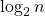.

Given a forbidden distance, we can form the restriction graph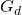with vertex setand edges between each pair of integers at distance.  This graph is a vertex-disjoint union of paths.  In particular, it is bipartite, so we can fix a bipartition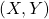ofso that all edges cross between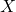and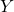.  Nowandare both legal choices for Claimer in this round, and at least one of them contains at least half of the uncovered points in.

### Lower bound

A lower bound is a strategy for Namer.  I’ll show that if Namer names the most common difference between the uncovered points ofthen the game will last at least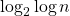rounds.

Suppose at some stage there are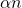points remaining.  There are only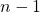possible distances between pairs of points in, so some distance occurs at least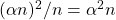times.  Thinking about the restriction graph on the uncovered points, by naming the most common distance Namer can ensure that there are at least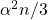uncovered points in the next round (the worst case being that the restriction graph consists of paths of length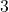).  Repeated squaring takes timeto reduce the density to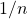(being deliberately vague about the base of the second logarithm).

### The truth

I don’t have a good conjecture for the true value.  Roughly speaking, the upper bound is correct if Namer can ensure that, at each stage, if there are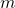points uncovered then some distance appears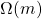times.  Conversely, the lower bound is correct if Claimer can ensure that each distance appears roughly equally often, for example by making the set of uncovered points look as random as possible.  But building this sort of random structure seems hard when we have less than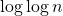time to do it.

Hereditarily non-uniform sets have been mentioned to me in connection with this problem, but haven’t enabled me to make any headway.